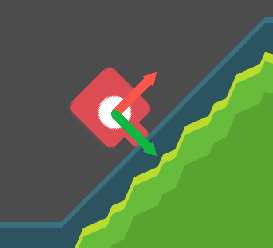# KinematicBody2D: align with surface

## Problem

You need your kinematic body to align with the surface it’s standing on.

## Solution

We’ll start with a basic kinematic platform character. See the Platform character recipe for details.

We have the following code for movement:

``````func _physics_process(delta):
get_input()
velocity.y += gravity * delta
velocity = move_and_slide(velocity, Vector2.UP, true)

if is_on_floor():
is_jumping = false
if Input.is_action_just_pressed("ui_up"):
is_jumping = true
velocity.y = jump_speed
``````

As you can see, there are a couple of problems. First, the character flies off the slope when running. It’s also sliding down the slope when there’s no input.

We can partially solve this by switching from `move_and_slide()` to `move_and_slide_with_snap()`:

``````snap = Vector2.DOWN * 128 if !is_jumping else Vector2.ZERO
velocity = move_and_slide_with_snap(velocity, snap, Vector2.UP, true)
``````

Now we have an upward “hop” when we stop on the way up the slope. This is because our `x` velocity is set to `0` by the lack of input, but the `y` is not.

## Orienting the velocity

We can fix this by orienting our velocity relative to the slope. To illustrate, let’s first rotate the character to align with the slope. We can do this by checking the floor normal when we’re on the floor:

``````if is_on_floor():
rotation = get_floor_normal().angle() + PI/2
``````

This hasn’t changed anything about the movement yet, but it does help us visualize what we need to do. When we’re on the slope, our local transform looks like this:Now when we move, we want our `x` velocity to align with our local `x` axis (the red arrow), and gravity/jump to align with local `y` (the green arrow). We can keep our input code the same, and just assume that `velocity` is always calculated in the local coordinate system. The only problem will be that `move_and_slide()` expects the velocity vector to be in global coordinates. Let’s adjust `move_and_slide_with_snap()` to account for this:

``````snap = transform.y * 128 if !is_jumping else Vector2.ZERO
velocity = move_and_slide_with_snap(velocity.rotated(rotation),
snap, -transform.y, true)
# Convert velocity back to local space.
velocity = velocity.rotated(-rotation)
``````

We’ve changed a few things here, so let’s look at them carefully.

• The `snap` vector is now our local down vector, so it will always point directly into the slope.
• The `floor_normal` parameter is also changed to the local up direction (`-transform.y`).
• We convert velocity to global by rotating it to match the player’s rotation, then revert the resulting velocity back to local by doing the reverse.

The result:

## Wrapping up

This technique allows for a wide range of possible platformer-style movement schemes. For example, you can do fun things like this:

Here’s the full script:

``````func _physics_process(delta):
get_input()
velocity.y += gravity * delta
snap = transform.y * 128 if !is_jumping else Vector2.ZERO
velocity = move_and_slide_with_snap(velocity.rotated(rotation),
snap, -transform.y, true, 4, PI/3)
velocity = velocity.rotated(-rotation)

if is_on_floor():
rotation = get_floor_normal().angle() + PI/2
is_jumping = false
if Input.is_action_just_pressed("ui_up"):
is_jumping = true
velocity.y = jump_speed
``````